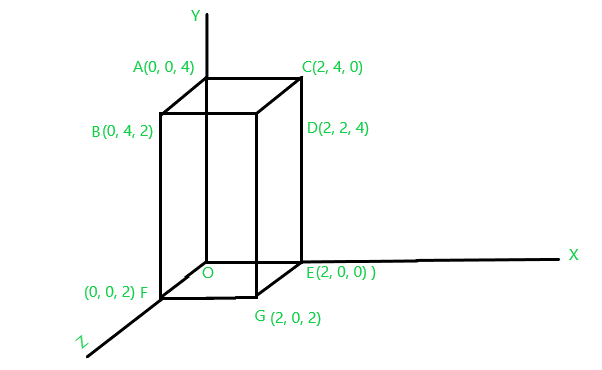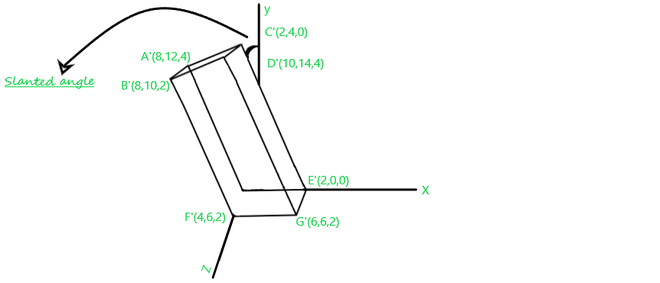Related Articles
Computer Graphics – 3D Shearing Transformation
• Last Updated : 14 Feb, 2021

Shearing transformation is the same as we see in 2D space, but here we have to deal with the x, y, and z axes whereas in 2D we deal with the only x and y axes. Shearing is the process of slanting an object in 3D space either in x, y, or in the z-direction. Shearing changes(or deformed) the shape of the object. AS we are discussing 3D space so shearing can also be done in any of the three directions as follows. Given below are the types of shearing transformation.

• Shearing in X-direction.
• Shearing in y-direction
• Shearing in z-direction.

Shearing in X-Direction: Here the coordinate of X remains unchanged while the coordinate of Y and Z is changed. Shearing is done through the Shearing Transformation matrix, which is represented as follows.Consider a point P[x, y, z] in 3D space over which we perform the shearing transformation in the X-direction and it becomes P'[x, y, z].Shearing in Y-Direction: Here the coordinate of Y remains unchanged while the coordinate of X and Z are changed. Shearing is done through the Shearing Transformation matrix, which is represented as follows.Consider a point P[x, y, z] in 3D space over which we perform the shearing transformation in the Y-direction and it becomes P'[x, y, z].Shearing in Z-Direction: Here the coordinate of Z remains unchanged while the coordinate of X and Y are changed. Shearing is done through the Shearing Transformation matrix, which is represented as follows for the shearing in Z-direction.Consider a point P[x, y, z] in 3D space over which we perform the shearing transformation in the Z-direction and its become P'[x, y, z].Note:  Here, xn, yn, zn Are  showing=new values and xo, yo, zo Are showing=old values.

Perform Shearing Transformation in the given cuboid(OABCDEFG) along Z-direction if a shearing parameter is as follows Sx=2, Sy=3.

The Shearing transformation matrix for z-direction is as follows.And the given cuboid is as below:Fig.1

Now, we’ll apply the shearing transformation condition over all the coordinates and calculate the new corresponding coordinates:

Point O[0, 0, 0] becomes O’ after performing Reflection transformation:Point A'[0, 0, 4] becomes A’ after performing Reflection transformation:Point B'[0, 0, 4] becomes B’ after performing Reflection transformation:Point C'[2, 4, 0] becomes C’ after performing Reflection transformation:Point D'[2, 2, 4] becomes D’ after performing Reflection transformation:Point E'[2, 0, 0] becomes E’ after performing Reflection transformation:Point F'[ 0, 0, 2] becomes F’ after performing Reflection transformation:Point G'[2, 0, 2] becomes G’ after performing Reflection transformation:Finally, after performing the Shearing transformation on the given cuboid your Fig.1 will look like as below:Fig.2

Attention reader! Don’t stop learning now. Get hold of all the important CS Theory concepts for SDE interviews with the CS Theory Course at a student-friendly price and become industry ready.

My Personal Notes arrow_drop_up